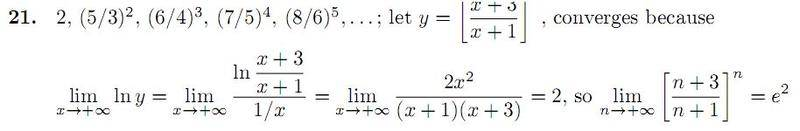# Natural Logs with Limits

Can someone explain to me how they get last line to work out?
I am confused on how they're using natural log with limit problems.

Thanks for the help!

:)

#### Attachments

•mathquestion1.JPG
15.3 KB · Views: 377

lanedance
Homework Helper
pics always take a while to clear, you;re better writing it up if you can

Didn't realize that. Sorry. :)Didn't realize that. Sorry. :)which equality in particular are you having a problem with? Or is it the introduction of the natural logarithm on the left-hand side?

I am having trouble with why he choose to let y what it does and how we went on from there.
How did he get

ln ((x+3)(x+1)/(1/x))

I am having trouble with why he choose to let y what it does and how we went on from there.
How did he get

ln ((x+3)(x+1)/(1/x))

This makes each term in the sequence y(x). It is also trivial that y(x) = eln(y(x)), which, in this case, is easier to study. He made use of the logarithm rule: ln(ax) = xln(a), and algebra: x = 1/(1/x), provided x is not 0.
Many limits are easily solved if you can find a clever rewriting of the algebraic expression like this.

How did he arrive at the 2x^2 / (x+3)(x+1) step? Is there something I'm missing he did when he took the limit to infinity?

How did he arrive at the 2x^2 / (x+3)(x+1) step? Is there something I'm missing he did when he took the limit to infinity?

The second limit gives us the indeterminate form 0/0, which makes it valid to use L'Hôpital's rule. This was the point of writing x as 1/(1/x).